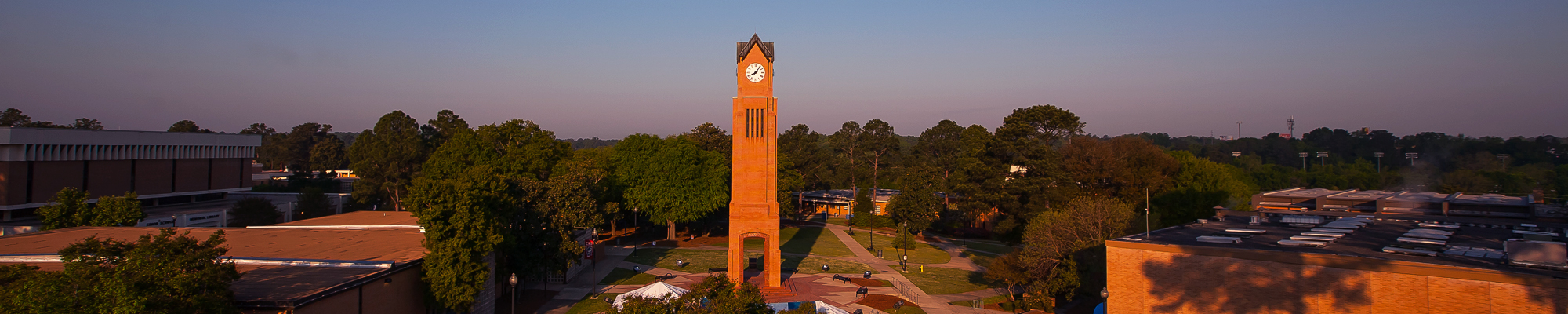## Mathematics Courses

MATH 1001. Quantitative Skills and Reasoning (3-0-3) This course is for students needing practical, comprehensive instruction, with a focus on life applications, college level study abilities, and clear understanding of mathematics for additional coursework, careers and everyday living. NOTE: This course is an alternative in Area A of the General Education Core Curriculum and is not intended to supply sufficient algebraic background for students who intend to take College Algebra, Pre-calculus, or Calculus. Students may not receive credit for both MATH 1001 and MATH 1101.

MATH 1101. Introduction to Mathematical Modeling (3-0-3) This course is an introduction to mathematical modeling using graphical, numerical, symbolic, and verbal techniques to describe and explore real-world data and phenomena. Emphasis is on the use of elementary functions to investigate and analyze applied problems and questions, supported by the use of appropriate technology, and on effective communication of quantitative concepts and results. NOTE: This course is an alternative in Area A of the General Education Core Curriculum and is not intended to supply sufficient algebraic background for students who intend to take College Algebra, Pre-calculus, or Calculus. Students may not receive credit for both MATH 1001 and MATH 1101.(Course fee required.)

MATH 1111. College Algebra (3-0-3) Prerequisite: Satisfactory Mathematics Placement. This course is a functional approach to algebra that incorporates the use of appropriate technology. Emphasis will be placed on the study of functions, and their graphs, inequalities, and linear, quadratic, piece-wise defined, rational, polynomial, exponential, and logarithmic functions. Appropriate applications will be included. Course is designed to develop algebraic concepts to a level sufficient for the study of calculus. (Course fee required.)

MATH 1113. Pre-Calculus (4-0-4) Prerequisite: MATH 1111 with a grade of "C" or better or an appropriate math placement score. This course is designed to prepare students for calculus, physics, and related technical subjects. Topics include algebraic and transcendental functions and an intensive study of trigonometric functions.

MATH 1125. Applied Calculus (3-0-3) Prerequisite: MATH 1111 with a grade of "C" or better, MATH 1113 with a grade of "C" or better, or an appropriate math placement score. Introduction to limits and continuity, differential calculus of algebraic, exponential, and logarithmic functions and integration. Applications in the fields of the behavioral, biological, and managerial sciences are included. (Course fee required.)

MATH 1131. Calculus with Analytic Geometry 1 (4-0-4) Prerequisite: MATH 1113 with a grade of "C" or better or an appropriate math placement score. Topics include exponential and logarithmic functions, introduction to limits and derivatives, computation and application of derivatives, and the definite integral. (Course fee required.)

MATH 1132. Calculus with Analytic Geometry 2 (4-0-4) Prerequisite: MATH 1131 with a grade of "C" or better. Topics include the definite and indefinite integrals, improper integrals, techniques of integration, applications of integration, and infinite sequences and series. (Course fee required.)

MATH 1165. Computer-Assisted Problem Solving (3-0-3) Prerequisites: MATH 1125 or MATH 1131 with a grade of "C" or better. Classes may be taken concurrently. Problem solving using contemporary technology such as graphing calculators, spread sheets, and computer algebra systems. Topics may include interpolation; linear regression; elementary differential models; and an introduction to the fundamentals of computer algebra systems, including manipulation of lists, sets, data structures, functions, plots, and program control structures. (Course fee required.)

MATH 2115. Introduction to Linear Algebra (3-0-3) Prerequisite: MATH 1131 may be taken concurrently. Systems of linear equations, matrix algebra, vector spaces, bases for a vector space, linear transformations, eigenvalues and eigenvectors, and matrix decompositions.

MATH 2125. Introduction to Discrete Mathematics (3-0-3) Prerequisite: MATH 1113 or MATH 1131 with a grade of "C" or better or a satisfactory math placement score. Topics include logic and proof, sets, functions, algorithms, the integers, matrices, mathematical reasoning, induction, recursion, counting, discrete probability, relations, graphs, trees, and Boolean algebra. (Course fee required.)

MATH 2135. Calculus with Analytic Geometry 3 (4-0-4) Prerequisite: MATH 1132 with a grade of "C" or better. Topics include parametric equations and polar coordinates, vectors, dot and cross products, vector functions of one real variable, real valued functions of several variables, differential calculus of functions of several variables, and multiple integrals.